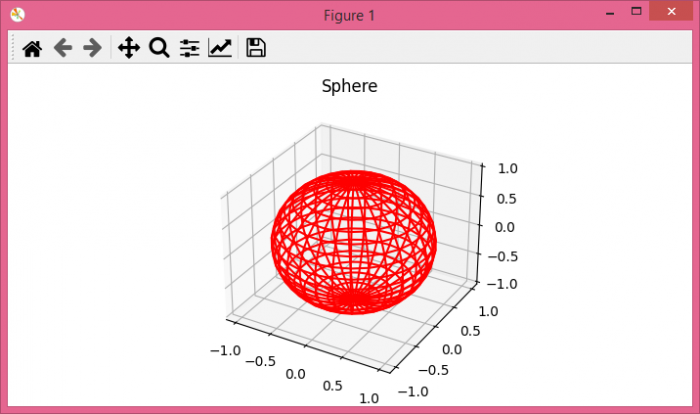# Saving a 3D-plot in a PDF 3D with Python

To save a 3D-plot in a PDF with Python, we can take the following steps

## Steps

• Set the figure size and adjust the padding between and around the subplots.

• Create a new figure or activate an existing figure.

• Add an 'ax' to the figure as part of a subplot arrangement.

• Create u, v, x, y and z data points using numpy.

• Plot a 3D wireframe.

• Set the title of the plot.

• Save the current figure using savefig() method.

## Example

import matplotlib.pyplot as plt
import numpy as np

plt.rcParams["figure.figsize"] = [7.00, 3.50]
plt.rcParams["figure.autolayout"] = True

fig = plt.figure()

u, v = np.mgrid[0:2 * np.pi:30j, 0:np.pi:20j]
x = np.cos(u) * np.sin(v)
y = np.sin(u) * np.sin(v)
z = np.cos(v)

ax.plot_wireframe(x, y, z, color="red")

ax.set_title("Sphere")

plt.savefig("test.pdf")

plt.show()


## Output

It will produce the following output −Also, it will create a PDF called test.pdf in the project directory and save this image in that file.